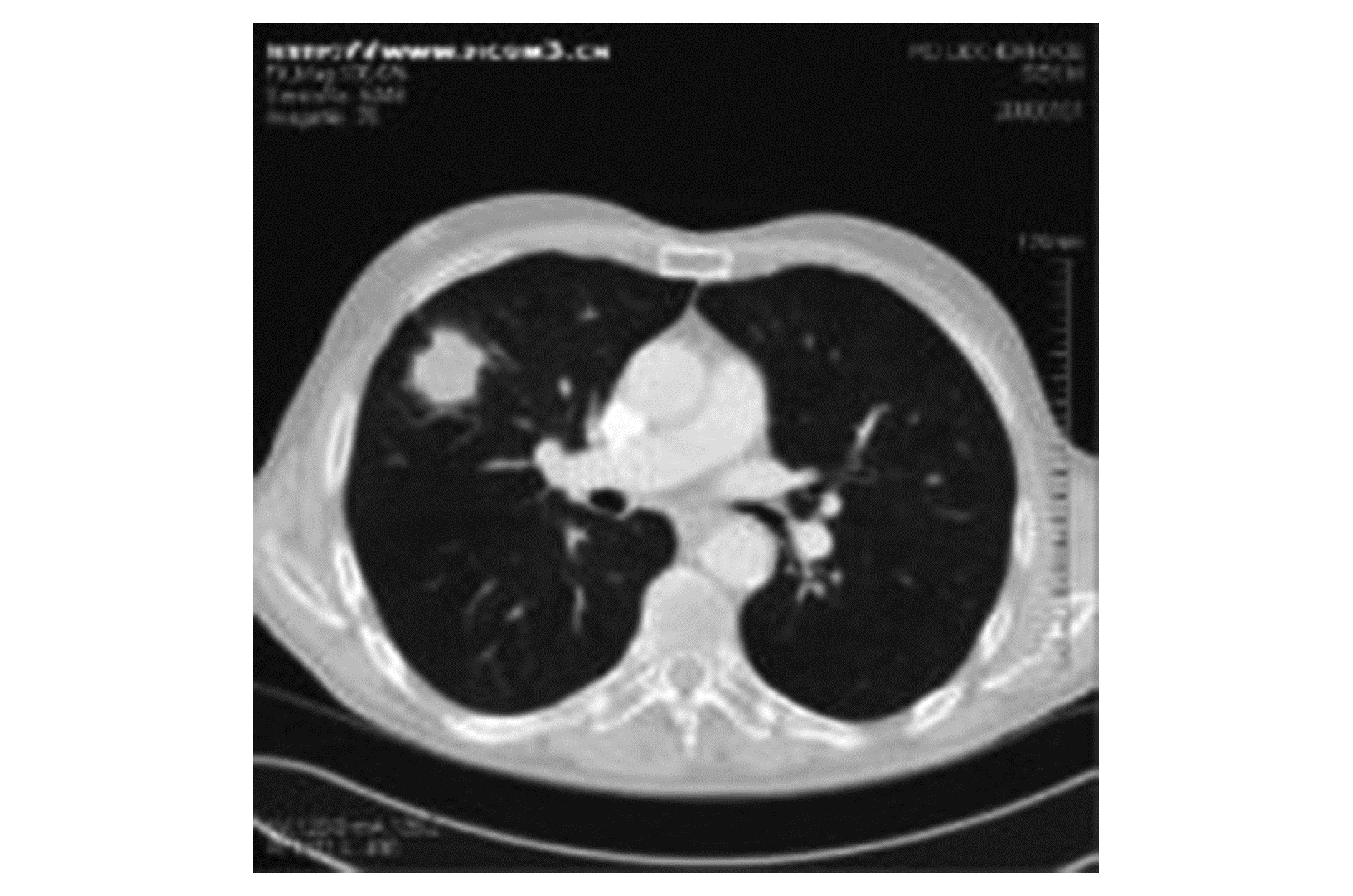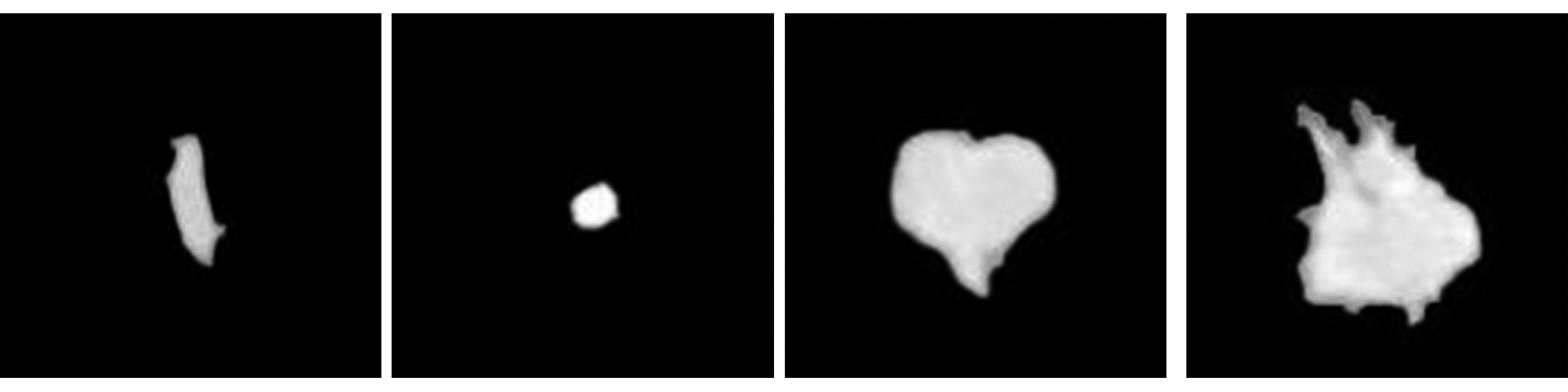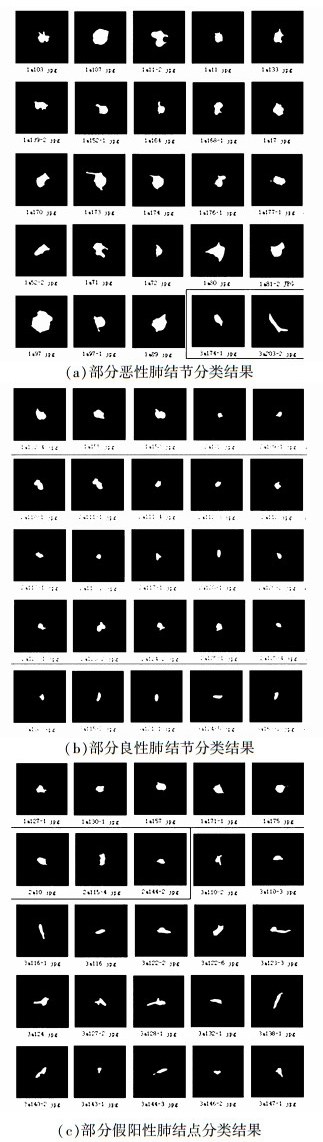﻿ 基于分布先验的半监督FCM的肺结节分类
«上一篇文章快速检索 高级检索

 智能系统学报2017, Vol. 12Issue (5): 729-734  DOI: 10.11992/tis.2017060180

### 引用本文JIANG Ting, XI Xiaoming, YUE Houguang. Classification of pulmonary nodules by semi-supervised FCM based on prior distribution[J]. CAAI Transactions on Intelligent Systems, 2017, 12(5): 729-734. DOI: 10.11992/tis.201706018.### 文章历史

Classification of pulmonary nodules by semi-supervised FCM based on prior distribution
JIANG Ting, XI Xiaoming, YUE Houguang
School of Computer Science and Technology, Shandong University of Finance and Economics, Ji'nan 250014, China
Abstract: The classification of pulmonary nodules is significant for the early detection and treatment of lung cancer. However, in real clinical applications, few medical images are labeled and it is difficult to obtain these labels. Semi-supervised learning methods that utilize supervised information to label images may be employed to improve the classification performance of pulmonary nodules. Traditional semi-supervised methods ignore the use of label information when the distribution between labeled and unlabeled specimens is imbalanced. To solve this problem, we propose a semi-supervised fuzzy c-means (FCM) algorithm based on prior distribution for classifying pulmonary nodules. This algorithm first calculates the prior probability of the specimens. Based on the obtained probability, a weight is assigned to each specimen for clustering to intensify the instruction role of a few labeled specimens in the clustering process. We conducted a corresponding test for Lung Image Database Consortium (LIDC) database. The result shows that, compared with the traditional semi-supervised FCM algorithm, the proposed algorithm can obtain better classification performances of pulmonary nodules.
Key words: classification of pulmonary nodules    semi-supervised FCM    prior distribution    image processing    LIDC database

1 特征提取图 1 LIDC中肺部CT图像 Fig.1 Lung CT image in LIDC图 2 肺结节分割 Fig.2 Segmentation of pulmonary nodules
2 肺结节的良恶性分类 2.1 经典FCM算法

FCM算法主要思想是在每个样本进行聚类时，引入一个类簇的隶属度计算样本属于某个类簇的可能性。聚类的过程可形式化为目标函数的优化过程，如式(1)所示。其中k的取值为式(2)所示。FCM算法通过不断迭代求解模糊隶属度函数uk和聚类中心vi，使得目标函数最小化，迭代停止，即完成聚类。

 ${J_m}\left( {u,v} \right) = \sum\limits_{i = 1}^C {\sum\limits_{k = 1}^N {u_{ik}^p{{\left\| {{\mathit{\boldsymbol{x}}_k} - {\mathit{\boldsymbol{v}}_i}} \right\|}^2}} }$ (1)
 $k = 1,2, \cdots ,N$ (2)
 ${u_{ik}} = \frac{1}{{\sum\limits_{j = 1}^n {{{\left( {\frac{{\left\| {{\mathit{\boldsymbol{x}}_k} - {\mathit{\boldsymbol{v}}_i}} \right\|}}{{\left\| {{\mathit{\boldsymbol{x}}_k} - {\mathit{\boldsymbol{v}}_j}} \right\|}}} \right)}^{\frac{2}{{p - 1}}}}} }}$ (3)
 ${v_i} = \frac{{\sum\limits_{k = 1}^N {u_{ik}^p{\mathit{\boldsymbol{x}}_k}} }}{{\sum\limits_{k = 1}^N {u_{ik}^p} }}$ (4)

2.2 部分监督的FCM算法

Bensaid在经典的FCM算法的基础上，提出了一种部分监督聚类算法。他提出的算法加强了标记信息在聚类过程中的指导作用。通过给标记样本赋予较大的权重，使标记样本在聚类中心的形成过程中发挥更重要的作用，提高了聚类的精度。其目标函数为

 ${J_m}\left( {u,v:x} \right) = \sum\limits_{i = 1}^C {\sum\limits_{k = 1}^N {u_{ik}^p\left\| {{\mathit{\boldsymbol{x}}_k} - {\mathit{\boldsymbol{v}}_i}} \right\|_A^2} }$ (5)

 ${v_{i,t}} = \frac{{\left( {\sum\limits_{k = 1}^n {{w_k}{{\left( {u_{ik,t}^d} \right)}^m}x_k^d} + \sum\limits_{k = 1}^n {{{\left( {u_{ik,t}^u} \right)}^m}x_k^u} } \right)}}{{\sum\limits_{k = 1}^n {{w_k}{{\left( {u_{ik,t}^d} \right)}^m}} + \sum\limits_{k = 1}^n {{{\left( {u_{ik,t}^u} \right)}^m}} }}$ (6)

 $u_{ik,t}^d = {f_{ik}}$ (7)
 $u_{ik,t}^u = \frac{1}{{\sum\limits_{j = 1}^n {{{\left( {\frac{{{{\left\| {\mathit{\boldsymbol{x}}_k^u - {\mathit{\boldsymbol{v}}_{i,t - 1}}} \right\|}_\mathit{\boldsymbol{A}}}}}{{{{\left\| {\mathit{\boldsymbol{x}}_k^u - {\mathit{\boldsymbol{v}}_{j,t - 1}}} \right\|}_\mathit{\boldsymbol{A}}}}}} \right)}^{\frac{2}{{m - 1}}}}} }}$ (8)

 $\left\| {{\mathit{\boldsymbol{x}}_K} - {\mathit{\boldsymbol{v}}_i}} \right\|_\mathit{\boldsymbol{A}}^2 = {\left( {{\mathit{\boldsymbol{x}}_k} - {\mathit{\boldsymbol{v}}_i}} \right)^{\rm{T}}}\mathit{\boldsymbol{A}}\left( {{\mathit{\boldsymbol{x}}_k} - {\mathit{\boldsymbol{v}}_i}} \right)$ (9)

A是任何的正定矩阵。

2.3 基于样本分布先验的半监督FCM算法

 $J = \sum\limits_{i = 1}^c {\sum\limits_{k = 1}^N {u_{ik}^pd_{ik}^2} } + \alpha \sum\limits_{i = 1}^c {\sum\limits_{k = 1}^N {\left( {{u_{ik}} - {f_{ik}}{b_k}} \right)d_{ik}^2} }$ (10)

 $q = M/N$ (11)
 $r = \left( {N - M} \right)/N$ (12)
 $\theta = 1 - q$ (13)
 $\beta = 1 - r$ (14)

 $u_{ik}^L = \theta \times \left( {\frac{1}{{1 + \alpha }}\left( {\frac{1}{{\sum\limits_{j = 1}^c {\frac{{d_{ik}^2}}{{d_{jk}^2}}} }} + \alpha {f_{ik}}} \right)} \right)$ (15)

 $u_{ik}^u = \beta \times \left( {\frac{1}{{\sum\limits_{j = 1}^c {\frac{{d_{ik}^2}}{{d_{jk}^2}}} }}} \right)$ (16)

 ${v_{ik}} = \frac{{\sum\limits_{{x_k} \in {x^d}} {{{\left( {\theta u_{ik}^L} \right)}^2}{x_k}} + \sum\limits_{{x_k} \in {x^u}} {{{\left( {\beta u_{ik}^u} \right)}^2}{x_k}} }}{{\sum\limits_{{x_k} \in {x^d}} {{{\left( {\theta u_{ik}^L} \right)}^2}} + \sum\limits_{{x_k} \in {x^u}} {{{\left( {\beta u_{ik}^u} \right)}^2}} }}$ (17)

1) 计算输入图像中肺结节的特征，并组成一个矩阵；

2) 确定样本个数C，平衡因子α，阈值ε，标记样本的先验概率q，未标记样本的先验概率r，以及标记样本的个数M

3) 初始化聚类中心vik以及模糊隶属度uik(包括标记样本的隶属度uikd以及未标记样本隶属度uiku)；

4) 按照式(15)、(16)更新模糊隶属度uik

5) 按照式(17)更新聚类中心；

6) 重复循环步骤3)，当两次迭代矩阵模糊隶属度的差小于ε阈值时停止迭代；

7) 根据计算得到的模糊隶属度uik结果进行分类。

3 实验结果及分析图 3 部分结节分类后的结果 Fig.3 Nodules classification results表 1 不同方法的准确率对比 Tab.1 Comparison of the accuracy of different methods

4 结束语

  MCGUIRE S. World Cancer Report 2014. Geneva, Switzerland:World Health Organization, International Agency for Research on Cancer, WHO Press, 2015[R]. Advances in nutrition, 2016, 7(2):418-419. (0)  伍长荣, 接标, 叶明全. CT图像肺结节计算机辅助检测与诊断技术研究综述[J]. 数据采集与处理, 2016, 31(5): 868-881. WU Changrong, JIE Biao, YE Mingquan. Reviews on computer-aided detection and diagnosis of pulmonary nodules in CT images[J]. Journal of data acquisition and processing, 2016, 31(5): 868-881. (0)  LEE S L A, KOUZANI A Z, HU E J. Automated detection of lung nodules in computed tomography images:a review[J]. Machine vision and applications, 2012, 23(1): 151-163. DOI:10.1007/s00138-010-0271-2 (0)  VALENTE I R S, CORTEZ P C, NETO E C, et al. Automatic 3D pulmonary nodule detection in CT images:A survey[J]. Computer methods and programs in biomedicine, 2016, 124(C): 91-107. (0)  HAN F, WANG H, ZHANG G, et al. Texture feature analysis for computer-aided diagnosis on pulmonary nodules[J]. Journal of digital imaging, 2015, 28(1): 99. DOI:10.1007/s10278-014-9718-8 (0)  HADY M F A, SCHWENKER F. Semi-supervised learning[J]. Intelligent systems reference library, 2010, 49(2): 215-239. (0)  BENSAID A M, HALL L O, BEZDEK J C, et al. Partially supervised clustering for image segmentation[J]. Pattern recognition, 1996, 29(5): 859-871. DOI:10.1016/0031-3203(95)00120-4 (0)  张慧哲, 王坚. 基于初始聚类中心选取的改进FCM聚类算法[J]. 计算机科学, 2009, 36(6): 206-209. ZHANG Huizhe, WANG Jian. Improved fuzzy C means clustering algorithm based on selecting initial clustering centers[J]. Computer science, 2009, 36(6): 206-209. (0)  李春芳, 庞雅静, 钱丽璞, 等. 半监督FCM聚类算法目标函数研究[J]. 计算机工程与应用, 2009, 45(14): 128-132. LI Chunfang, PANG Yajing, QIAN Lipu, et al. Objective function of semi-supervised FCM clustering algorithm[J]. Computer engineering and application, 2009, 45(14): 128-132. DOI:10.3778/j.issn.1002-8331.2009.14.039 (0)  WU K L. Analysis of parameter selections for fuzzy C-means[J]. Pattern recognition, 2012, 45(1): 407-415. DOI:10.1016/j.patcog.2011.07.012 (0)  侯薇, 董红斌, 印桂生. 一种基于隶属度优化的演化聚类算法[J]. 计算机研究与发展, 2013, 50(3): 548-558. HOU Wei, DONG Hongbin, YIN Guisheng. A membership degree refinement-based evolutionary clustering algorithm[J]. Journal of computer research and development, 2013, 50(3): 548-558. DOI:10.7544/issn1000-1239.2013.20111346 (0)  李斌, 狄岚, 王少华, 等. 基于改进核模糊C均值类间极大化聚类算法[J]. 计算机应用, 2016, 36(7): 1981-1987. LI Bin, DI Lan, WANG Shaohua, et al. Clustering algorithm with maximum distance between clusters based on improved kernel fuzzy C-means[J]. Journal of computer applications, 2016, 36(7): 1981-1987. DOI:10.11772/j.issn.1001-9081.2016.07.1981 (0)  文传军, 汪庆淼, 詹永照. 均衡模糊C均值聚类算法[J]. 计算机科学, 2014, 41(8): 250-253. WEN Chuanjun, WANG Qingmiao, ZHAN Yongzhao, et al. Equalization fuzzy C-means clustering algorithm[J]. Computer science, 2014, 41(8): 250-253. DOI:10.11896/j.issn.1002-137X.2014.08.053 (0)  蔡加欣, 杨丰, 冯国灿. 改进退化的半监督模糊聚类应用于MR图像分割[J]. 中国图象图形学报, 2011, 16(5): 784-791. CAI Jiaxin, YANG Feng, FENG Guocan, et al. Degeneracy-improved semi-supervised fuzzy clustering with application in MR image segmentation[J]. Journal of image and graphics, 2011, 16(5): 784-791. DOI:10.11834/jig.20110521 (0)  苏志远, 刘慧, 尹义龙. 基于弱监督ECOC算法的肺结节辅助检测[J]. 数据采集与处理, 2015, 30(5): 1003-1010. SU Zhiyuan, LIU Hui, YIN Yilong, et al. Pulmonary nodule aided detection based on weakly-supervised ECOC algorithm[J]. Jornal of data acqusition and processing, 2015, 30(5): 1003-1010. (0)  MURRAY P, MARSHALL S. A new design tool for feature extraction in noisy images based on grayscale hit-or-miss transforms[J]. IEEE transactions on image processing a publication of the ieee signal processing society, 2011, 20(7): 1938-48. DOI:10.1109/TIP.2010.2103952 (0)  BAE H J, KANG E Y, YONG H S, et al. Paratracheal air cysts on thoracic multidetector CT:incidence, morphological characteristics and relevance to pulmonary emphysema[J]. British journal of radiology, 2013, 86(1021): 20120218. DOI:10.1259/bjr.20120218 (0)  BEZDEK J C, EHRLICH R, FULL W. FCM:the fuzzy C-means clustering algorithm[J]. Computers and geosciences, 1984, 10(2/3): 191-203. (0)  PEDRYCZ W, WEMBER J, WALETZKY J. Fuzzy clusteringwith partial supervision[J]. IEEE transaction on system, man, and cybernetics, part B:cybernetics, 1997, 27(5): 787-795. DOI:10.1109/3477.623232 (0)  MCNITT-GRAY M F, MEYER C R, REEVES A P, et al. The lung image database consortium (LIDC) data collection process for nodule detection and annotation[J]. Academic radiology, 2008, 14(12): 1464-1474. (0)  李秋萍, 刘慧, 苏志远. 基于改进的半监督FCM聚类算法的肺结节分类与识别[J]. 图学学报, 2015, 36(2): 244-250. LI Qiuping, LIU Hui, SU Zhiyuan. Modified fuzzy clustering with partial supervision algorithm in classification and recognition of pulmonary nodules[J]. Journal of graphics, 2015, 36(2): 244-250. (0)